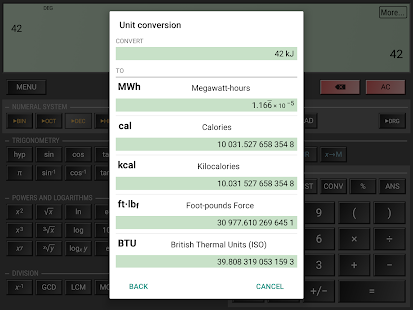HiPER Calc Pro is an advanced version of HiPER Scientific Calculator. The calculator has up to 100 digits of significand and 9 digits of exponent. It detects repeating decimals and numbers in it can be also entered as fractions or converted to fractions. You can also switch to the “expression” mode where you can write expressions in a natural way and watch your calculations. When possible, an expression is simplified and the result is displayed as an expression using fractions, square roots and ? constant. The multiline display can be turned on in tablets to show the complete history of calculations and to provide access the previous results. The users can choose from several high-quality themes.

The calculator has several functions, such as:

• basic arithmetic operations including percentage, modulo and negation;
• fractions (in the expression mode any expression including nested fractions can be entered as a numerator and a denominator);
• mixed numbers;
• periodic numbers and their conversion to fractions;
• unlimited number of braces;
• operator priority;
• repeated operations;
• calculation details – extended information about a calculation like all complex roots, unit circle etc.;
• complex numbers
• conversion between rectangular and polar coordinates
• advanced number operations such as random numbers, combinations, permutations, common greatest divisor, etc.;
• trigonometric and hyperbolic functions;
• Powers, roots, logarithms, etc.;
• degrees, minutes and seconds conversion;
• fixed point, scientific and engineering display format;
• display exponent as SI units prefix;
• memory operations with 10 extended memories;
• clipboard operations with various clipboard formats;
• result history;
• binary, octal and hexadecimal numeral systems;
• logical operations;
• bitwise shifts and rotations;
• haptic feedback;
• more than 90 physical constants;
• conversion among 200 units;
• Reverse Polish notation.

Screenshots

•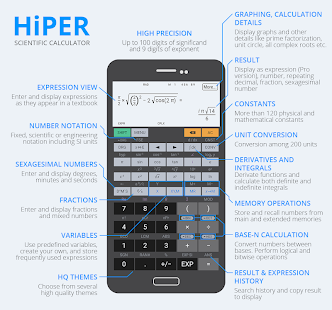•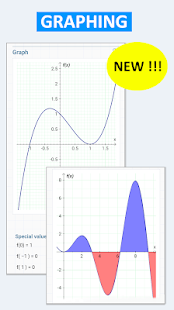•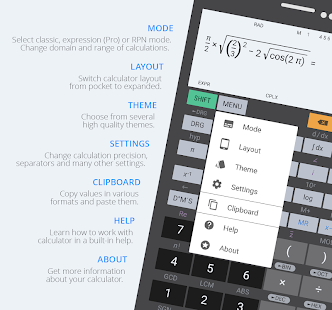•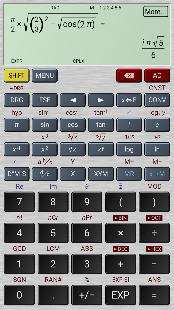•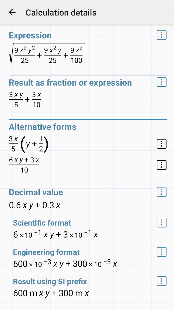•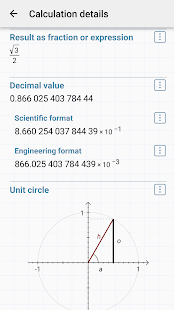•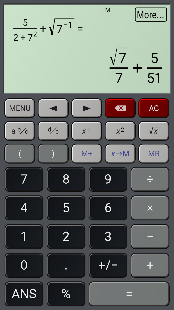•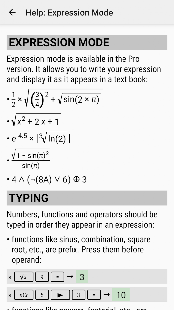••••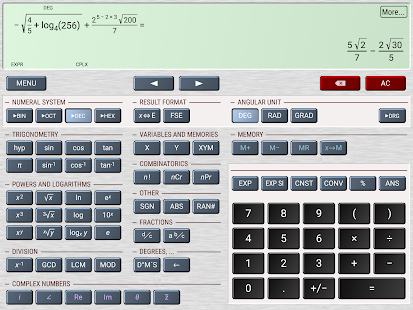•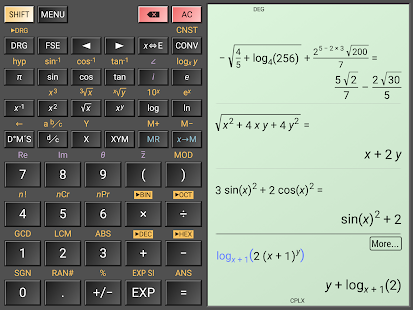•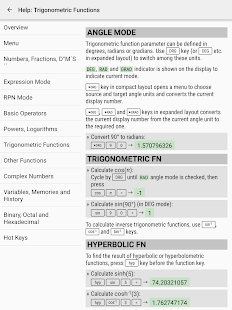•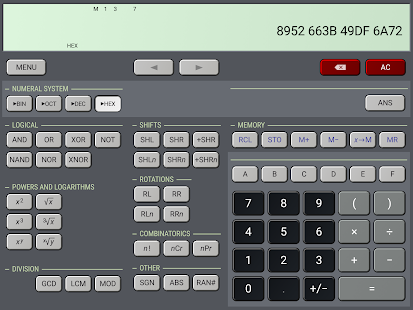•